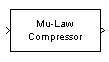Documentation

Mu-Law Compressor

Implement µ-law compressor for source coding

Library

Source CodingDescription

The Mu-Law Compressor block implements a µ-law compressor for the input signal. The formula for the µ-law compressor is

$y=\frac{V\mathrm{log}\left(1+\mu |x|/V\right)}{\mathrm{log}\left(1+\mu \right)}\mathrm{sgn}\left(x\right)$

where µ is the µ-law parameter of the compressor, V is the peak magnitude of x, log is the natural logarithm, and sgn is the signum function (sign in MATLAB®).

The input can have any shape or frame status. This block processes each vector element independently.

Parameters

mu value

The µ-law parameter of the compressor.

Peak signal magnitude

The peak value of the input signal. This is also the peak value of the output.

Supported Data Type

PortSupported Data Types
In
• double

Out
• double

Mu-Law Expander

References

 Sklar, Bernard. Digital Communications: Fundamentals and Applications. Englewood Cliffs, N.J.: Prentice-Hall, 1988.